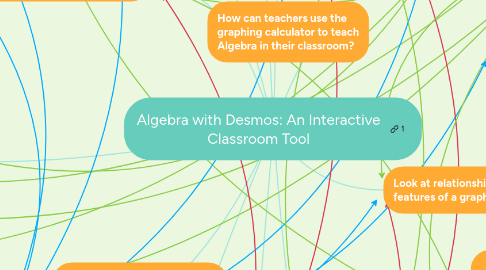# Algebra with Desmos: An Interactive Classroom Tool

Get Started. It's FreeAlgebra with Desmos: An Interactive Classroom Tool## 1. Graphing

### 1.2. Highlight key features of the graph

1.2.1. These features may include x and y intercepts, maxima and minima, and asymptotes.

## 2. Look at relationships between features of a graph

### 2.1. "Sliders" allow students to change values of standard form equations

2.1.1. This helps students see what each portion of a standard form equation represents

2.1.2. Teachers can ask students guiding questions to help them realize what the variables mean

## 5. Assessment of Student Learning

### 5.2. Students have to answer questions as they move through the presentation

5.2.1. Easily see student answers and contributions

5.2.2. Teacher gets to decide whether or not students can see the contributions their classmates made to the lesson!

## 8. What standards is Desmos a tool for?

### 8.1. Ratios and Proportions

8.1.1.1. Learning to graph on a coordinate plane.

8.1.2.1. Explaining the relationship between two plotted values.

### 8.2. Expressions, Equations, and Inequalities

8.2.1.1. Graph the solution of the inequality.

8.2.2.1. Write and graph inequalities. Desmos has a built-in feature to help students write inequalities and to see the solution set of the problem.

8.2.3.1. Graph proportional relationships.